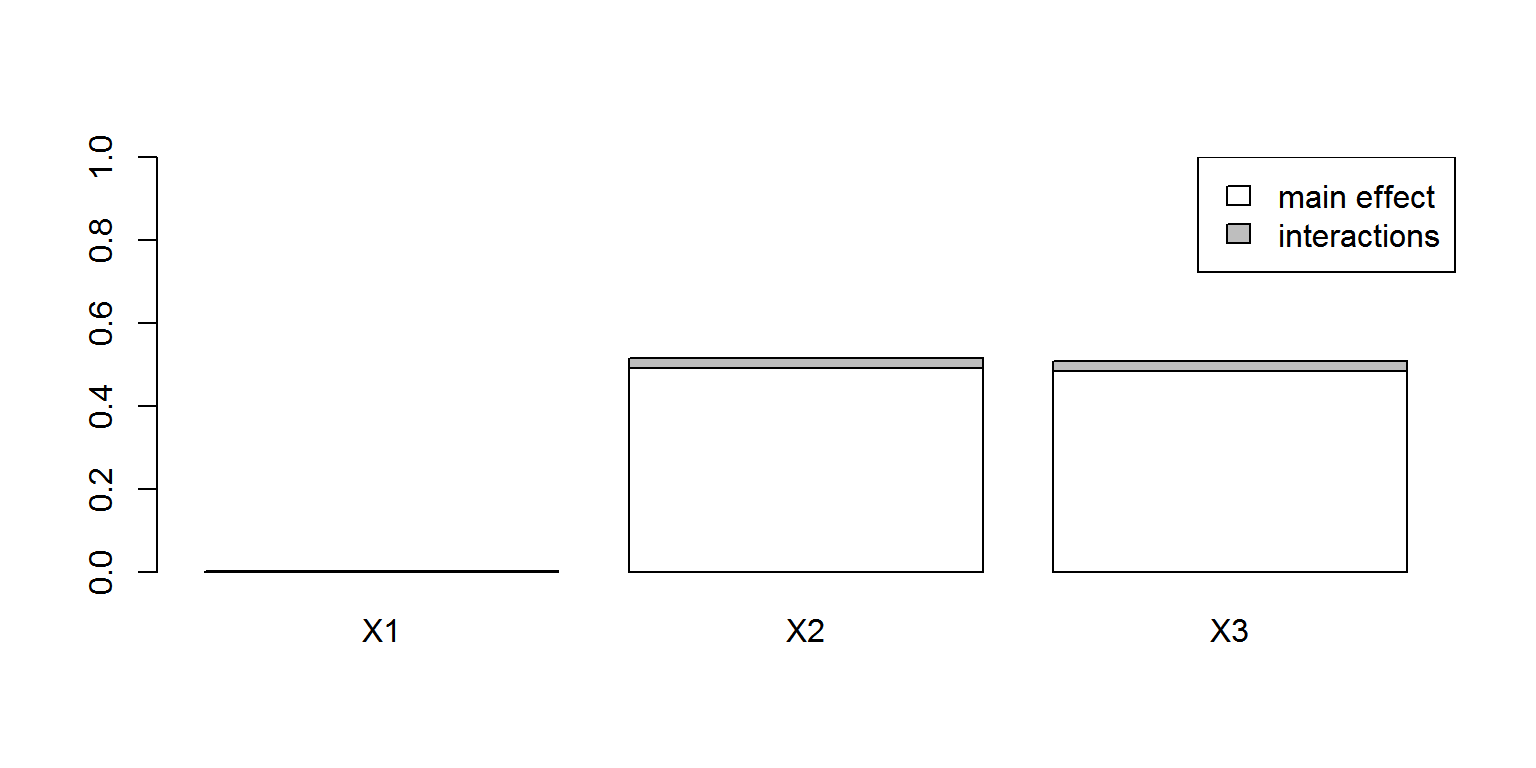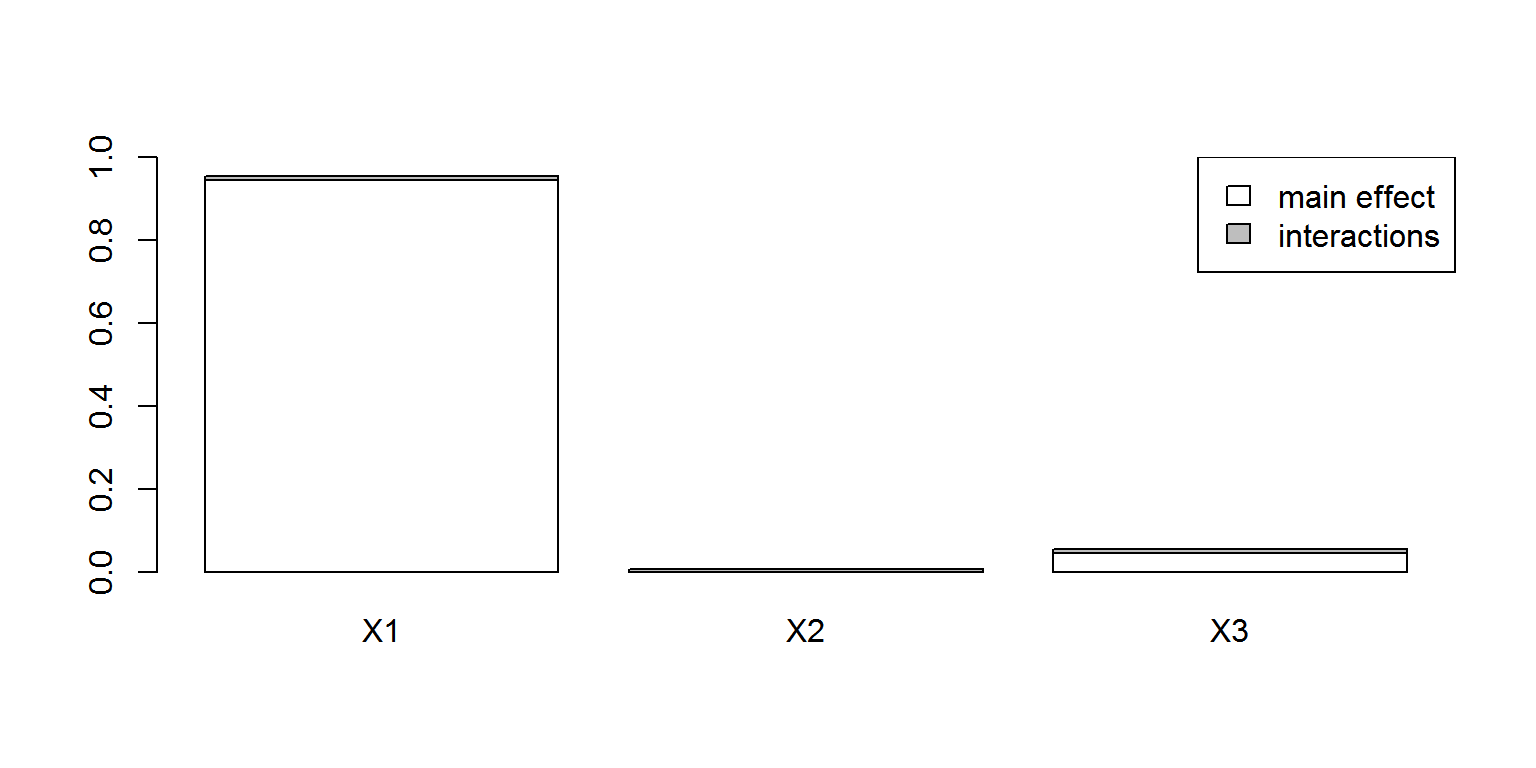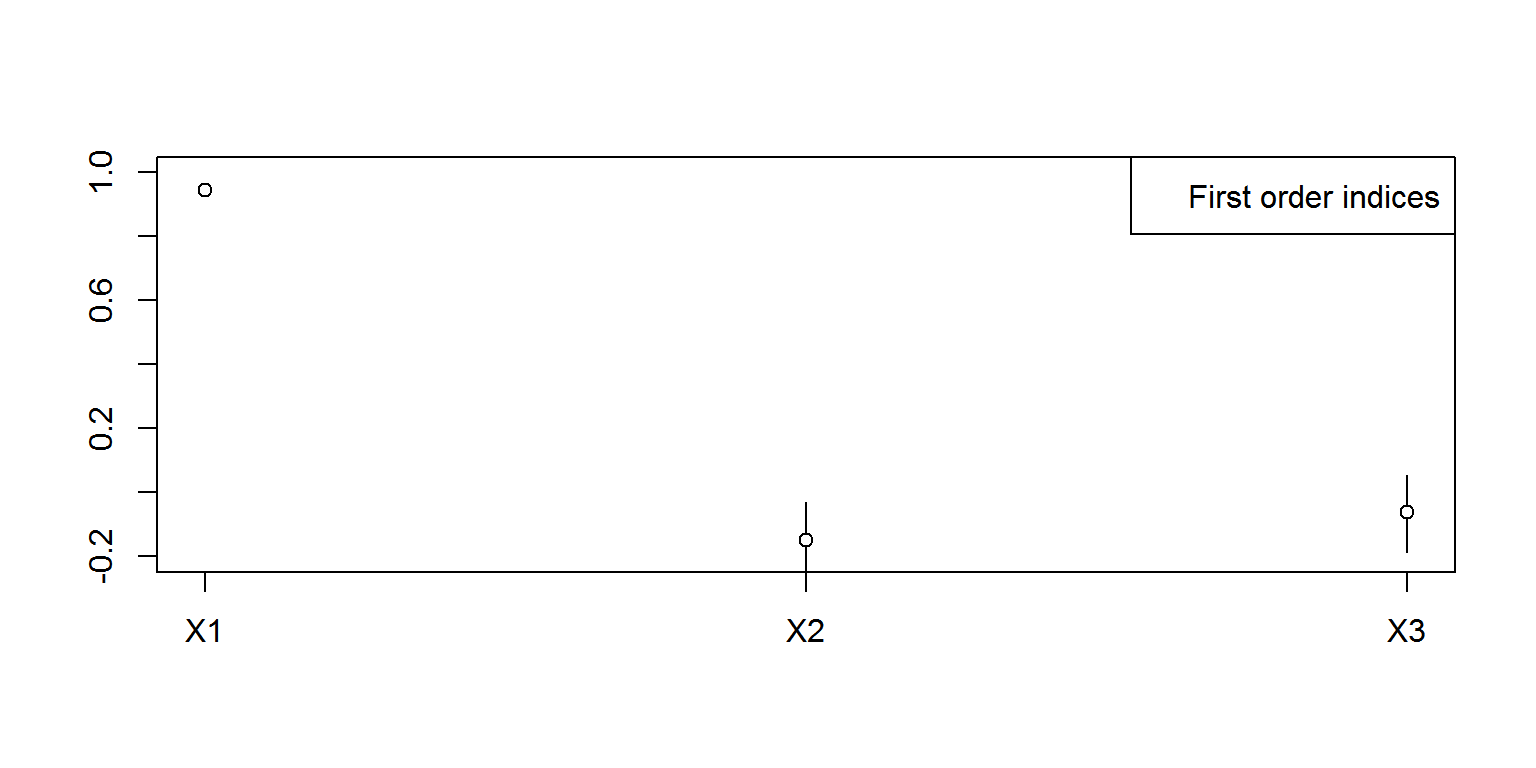$$\newcommand{\esp}{\mathbb{E}\left(#1\right)} \newcommand{\var}{\mbox{Var}\left(#1\right)} \newcommand{\deriv}{\dot{#1}(t)} \newcommand{\prob}{ \mathbb{P}\!(#1)} \newcommand{\eqdef}{\mathop{=}\limits^{\mathrm{def}}} \newcommand{\by}{\boldsymbol{y}} \newcommand{\bc}{\boldsymbol{c}} \newcommand{\bpsi}{\boldsymbol{\psi}} \def\pmacro{\texttt{p}} \def\like{{\cal L}} \def\llike{{\cal LL}} \def\logit{{\rm logit}} \def\probit{{\rm probit}} \def\one{{\rm 1\!I}} \def\iid{\mathop{\sim}_{\rm i.i.d.}} \def\simh0{\mathop{\sim}_{H_0}} \def\df{\texttt{df}} \def\res{e} \def\xomega{x} \newcommand{\argmin}{{\rm arg}\min_{#1}} \newcommand{\argmax}{{\rm arg}\max_{#1}} \newcommand{\Rset}{\mbox{\mathbb{R}}} \def\param{\theta} \def\setparam{\Theta} \def\xnew{x_{\rm new}} \def\fnew{f_{\rm new}} \def\ynew{y_{\rm new}} \def\nnew{n_{\rm new}} \def\enew{e_{\rm new}} \def\Xnew{X_{\rm new}} \def\hfnew{\widehat{\fnew}} \def\degree{m} \def\nbeta{d} \newcommand{\limite}{\mathop{\longrightarrow}\limits_{#1}} \def\ka{k{a}} \def\ska{k{\scriptscriptstyle a}} \def\kel{k{e}} \def\skel{k{\scriptscriptstyle e}} \def\cl{C{\small l}} \def\Tlag{T\hspace{-0.1em}{lag}} \def\sTlag{T\hspace{-0.07em}{\scriptscriptstyle lag}} \def\Tk{T\hspace{-0.1em}{k0}} \def\sTk{T\hspace{-0.07em}{\scriptscriptstyle k0}} \def\thalf{t{1/2}} \newcommand{\Dphi}{\partial_\pphi #1} \def\asigma{a} \def\pphi{\psi} \newcommand{\stheta}{{\theta^\star}} \newcommand{\htheta}{{\widehat{\theta}}}$$

For complex PKPD models it can be difficult to understand the contribution of each parameter to the model outputs. In such cases a sensitivity analysis can be a powerful way to investigate which parameter will have the biggest influence.

A sensitivity analysis can be performed with the R package sensitivity.

Sensitivity analysis for longitudinal data should be based on an unidimensional summary of the data, such as the integral, i.e. the area under the curve (AUC), the minimum or the maximum value over an interval, for instance. Such quantities can easily be computed with the function exposure of the mlxR package.

Combining the packages mlxR and sensistivity is therefore a powerful solution for sensitivity analysis of PKPD models.

library(sensitivity)

We will consider a basic one-compartement PK model for oral administration. The sensitivity analysis will be based on the exposure at steady state, when repeated doses of 5 mg are given every 12 hours.

The model and the design are defined in a function which returns either AUC, Cmax or Tmax according to the quantity used for the sensitivity analysis.

The PK parameters $$ka$$, $$V$$ and $$k$$ are the input arguments of this function.

sensModel <- function(x){

myModel <- inlineModel("
[LONGITUDINAL]
input = {ka, V, k}
EQUATION:
Cc = pkmodel(ka, V, k)
")

N <- dim(x)
x <- cbind((1:N),x)
p <- list(name=c("ka", "V", "k"),
colNames=c("id", "ka", "V", "k"),
value=x)

res <- exposure(model     = myModel,
parameter = p,
treatment = list(tfd=0, ii=12, amount=5))

# For computing the exposure during the first dose interval, set
#  output = list(name="Cc", time=c(0,12, by=100)),
#  treatment = list(time=0, amount=5),

if (i.out=="tmax"){
r <- res$Cc$tmax
}else if(i.out=="cmax"){
r <- res$Cc$cmax
}else if(i.out=="auc"){
r <- res$Cc$auc
}else if(i.out=="all"){
r <- res$Cc } return(r) } If we want to see, for instance, the impact of $$ka$$ on the exposure at steady state, we can use this function with two different sets of PK parameters ($$ka=0.2$$, $$V=10$$, $$k=0.2$$ and $$ka=0.5$$, $$V=10$$, $$k=0.2$$) and return the output of exposure by setting i.out to “all”. i.out <- "all" r <- sensModel(matrix(c(0.2,0.5,10,10,0.2,0.2),nrow=2)) print(r) ## id t1 t2 step auc tmax cmax tmin cmin ## 1 1 36 48 0.1212121 2.498084 39.75757 0.2568232 36 0.13130065 ## 2 2 36 48 0.1212121 2.499413 38.78788 0.3174729 36 0.08100781 We can see that changing the value of $$ka$$ from 0.2 to 0.5 doesn’t modify the area under the curve (auc) but impacts the time at which the maximum concentration is observed (tmax). For a more exhaustive sensitivity analysis, let us use now the fast99 function which implements the so-called extended-FAST method (Saltelli et al. 1999). Here, $$ka$$ takes its values between 0.2 and 0.5, $$V$$ between $$5$$ and $$10$$, and $$k$$ between 0.05 and 0.1. Sensitivity analysis is first based on $$AUC$$. l1 <- list(min = 0.2, max = 0.5) l2 <- list(min = 5, max = 10) l3 <- list(min = 0.05, max = 0.1) i.out <- "auc" x <- fast99(model = sensModel, factors = 3, n = 500, q = "qunif", q.arg = list(l1,l2,l3) ) print(x) ## ## Call: ## fast99(model = sensModel, factors = 3, n = 500, q = "qunif", q.arg = list(l1, l2, l3)) ## ## Model runs: 1500 ## ## Estimations of the indices: ## first order total order ## X1 2.217184e-06 0.002148368 ## X2 4.911908e-01 0.515163373 ## X3 4.846578e-01 0.508640147 plot(x)Sobol indices measure the contributions of each PK parameter to the variability of $$AUC$$. A Sobol index of zero means that the variance of the parameter has no contribution on the variance of the output and a Sobol index of one means that the variance in the output is 100% dependent on the variance of the parameter. This index is almost 0 for $$ka$$ but is significantly different from 0 for both $$V$$ and $$k$$ If we now use $$Tmax$$ instead of $$AUC$$ for our sensitivity analysis, we see that it’s $$ka$$ which explains almost all the variability of $$Tmax$$. i.out <- "tmax" x <- fast99(model = sensModel, factors = 3, n = 500, q = "qunif", q.arg = list(l1,l2,l3) ) print(x) ## ## Call: ## fast99(model = sensModel, factors = 3, n = 500, q = "qunif", q.arg = list(l1, l2, l3)) ## ## Model runs: 1500 ## ## Estimations of the indices: ## first order total order ## X1 9.437639e-01 0.955043814 ## X2 2.508492e-05 0.005986033 ## X3 4.422586e-02 0.053724432 plot(x)Several methods are available in the package sensitivity. sobolEff implements the Monte Carlo estimation of the Sobol’ sensitivity indices using the asymptotically efficient formulas in section 4.2.4.2 of Monod et al. (2006). We need a function that randomly draws PK parameters uniformly distributed in given intervals. mySim <- function(r,n){ M <- length(r) X <- data.frame(matrix(runif(M * n), nrow = n)) for (m in (1:M)){ rm <- r[[m]] X[,m] <- X[,m]*(rm$max-rm$min) + rm$min
}
return(X)
}

Monte Carlo methods for estimating the Sobol indices use two random samples $$X1$$ and $$X2$$ of the PK parameters.

X1 <- mySim(list(l1,l2,l3),n=200)
X2 <- mySim(list(l1,l2,l3),n=200)
x <- sobolEff(model=sensModel, X1=X1, X2=X2, order=1, nboot=500)
print(x)
##
## Call:
## sobolEff(model = sensModel, X1 = X1, X2 = X2, order = 1, nboot = 500)
##
## Model runs: 800
##
## Model variance: 0.1311307
##
##
##
## Sobol indices
##       original          bias  std. error  min. c.i.   max. c.i.
## X1  0.94402401 -2.189272e-05 0.006345276  0.9330730  0.95678564
## X2 -0.14805342  7.382559e-04 0.063559259 -0.2658611 -0.02910147
## X3 -0.06181223 -5.239164e-04 0.064811691 -0.1853228  0.05392660
plot(x, ylim=c(-0.2,1))[Package “sensitivity”] (http://cran.r-project.org/web/packages/sensitivity/sensitivity.pdf)

A. Saltelli, S. Tarantola and K. Chan, 1999, A quantitative, model independent method for global sensitivity analysis of model output, Technometrics, 41, 39-56.

Monod, H., Naud, C., Makowski, D. (2006), Uncertainty and sensitivity analysis for crop models in Working with Dynamic Crop Models: Evaluation, Analysis, Parameterization, and Applications, Elsevier.